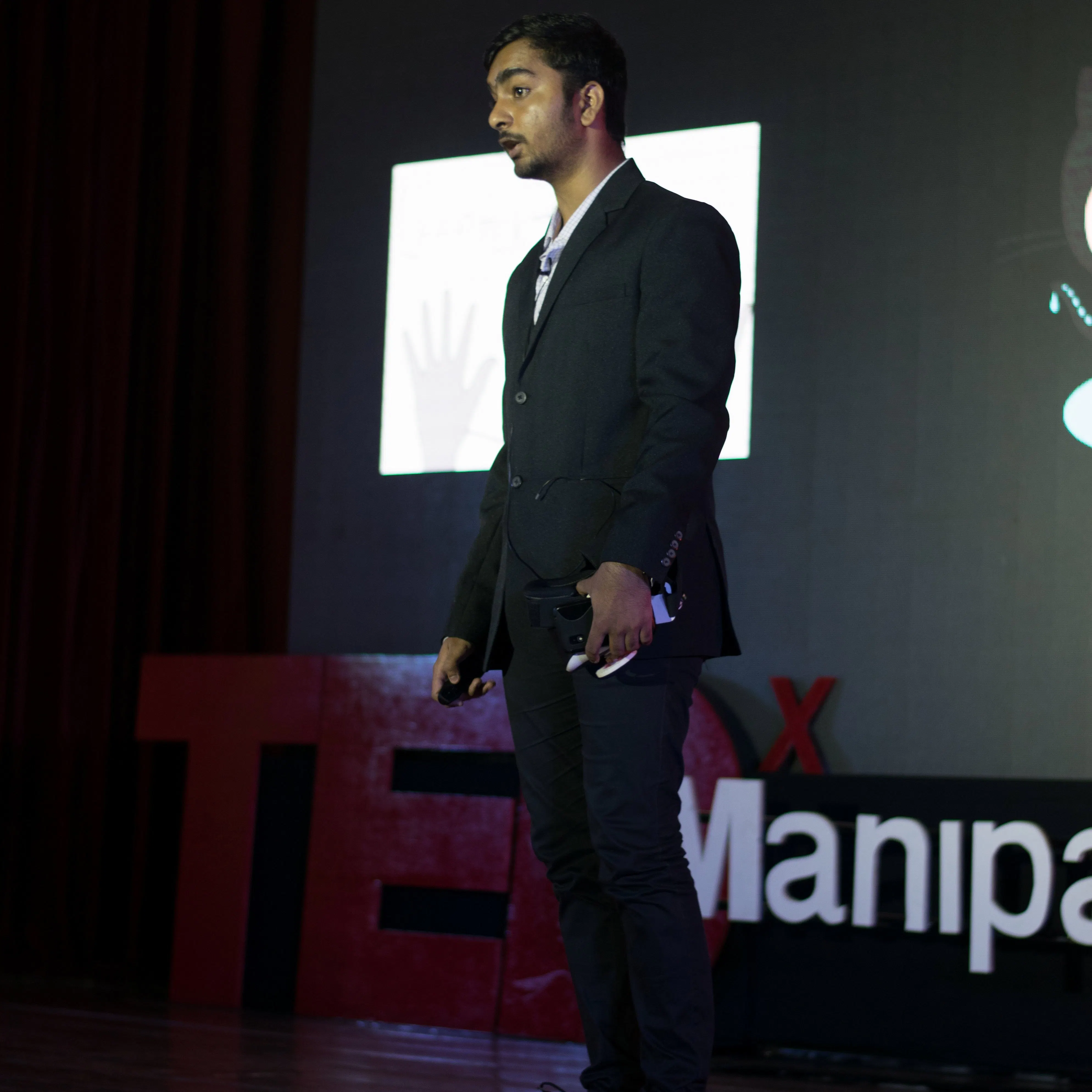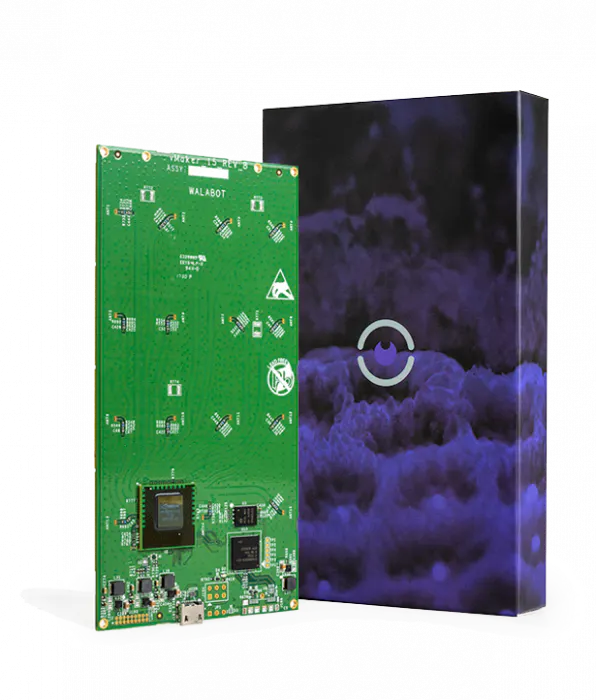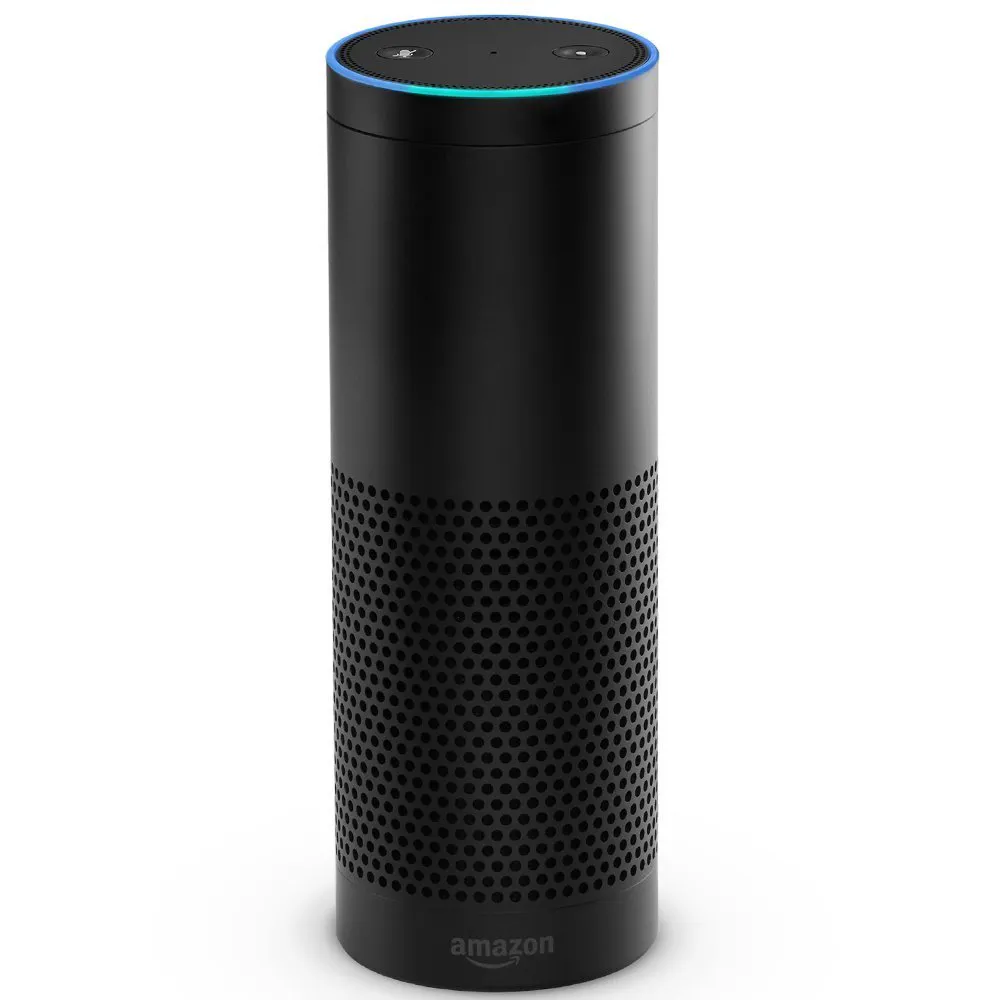Published © MIT

# Curismo: A Smart Pill Box Assistant For Users With Dementia

Curismo uses Walabot to detect when a user picks up a pill and a custom Alexa skill to help user keep track of their daily pill intake.

IntermediateFull instructions provided1,827## Things used in this project

### Hardware componentsWalabot Creator
×1Amazon Alexa Amazon Echo
×1

### Software apps and online servicesAmazon Alexa Alexa Skills Kit
 Flask-Ask - Python Alexa Skills Development Library

Read more

## Code

### curismoalexa.py

Python
Alexa Skill written using Flask-Ask
```"""
Curismo : A Smart Pill Box Assistant
Developer : Geeve George
External Libraries used : Flask-Ask
"""

import logging
from random import randint
from flask import Flask, render_template #library for building skills using python
from flask_ask import Ask, statement, question, session

from dateutil.parser import parse #parse time corresponding to pill intake logged into datatime.txt file by walabot
import re
from datetime import datetime

p1 = 0
p2 = 0
p3 = 0
v = 0
i =0

match = 0
p1time = 0
p2time = 0
p3time = 0

prescp_p1 = 3
prescp_p2 = 3
prescp_p3 = 3

def readpilltiming():
global p1,p2,p3,v,i,match,p1time,p2time,p3time
with open('datatime.txt', 'r') as f: #read file that contains information logged by walabot

while True:
if(i==3):
i = 0
if(i<3):
v = f.readline() #extract time substring from each line
if not v: break

if("lm : " in v):
match = parse(v, fuzzy=True)
p1time = datetime.strptime(str(match.hour)+':'+str(match.minute), '%H:%M').strftime("%I:%M %p") #convert to 24 hour to 12 hour format

elif("mm : " in v):
match = parse(v, fuzzy=True)
p2time = datetime.strptime(str(match.hour)+':'+str(match.minute), '%H:%M').strftime("%I:%M %p")

elif("rm : " in v):
match = parse(v, fuzzy=True)
p3time = datetime.strptime(str(match.hour)+':'+str(match.minute), '%H:%M').strftime("%I:%M %p")

f.close()

def readnupdate():
global p1,p2,p3,v,i
with open('data.txt', 'r') as f:

while True:
if(i==3):
i = 0
if(i<3):
v = f.readline()
if not v: break

if("lm : " in v): #extract pill intake count
[int(s) for s in v.split() if s.isdigit()]
p1 = int(s)

elif("mm : " in v):
[int(s) for s in v.split() if s.isdigit()]
p2 = int(s)

elif("rm : " in v):
[int(s) for s in v.split() if s.isdigit()]
p3 = int(s)

f.close()

app = Flask(__name__)
ask = Ask(app, "/")
logging.getLogger("flask_ask").setLevel(logging.DEBUG)

@ask.launch
def starting():
readnupdate()
welcome = render_template('welcome')
return statement(welcome)

@ask.intent("AllPillIntent")
def allpill():
readnupdate()
global p1,p2,p3
return statement('as of now, you have had {} pill 1, {} pill 2 and {} pill 3'.format(p1,p2,p3))

@ask.intent("DailyPrescpIntent")
def dailypres():
readnupdate()
return statement('As per your prescription, you need to have {} pill 1 with food, {} pill 2 and {} pill 3 without daily. As of now you have had {} pill 1, {} pill 2 and {} pill 3'.format(prescp_p1,prescp_p2,prescp_p3,p1,p2,p3))

@ask.intent("PillNotTakenIntent")
def pillnothad():
readnupdate()
global p1,p2,p3
if(p1==0 and p2==0 and p3==0):
return statement('you have not had any pills today')
elif(p1==0 and p2==0 and p3!=0):
return statement('you have not had pill 1 and pill 2')
elif(p1==0 and p2!=0 and p3==0):
return statement('you have not had pill 1 and pill 3')
elif(p1!=0 and p2==0 and p3==0):
return statement('you have not had pill 2 and pill 3')
elif(p1==0 and p2!=0 and p3!=0):
return statement('you have not had pill 1 today')
elif(p1!=0 and p2==0 and p3!=0):
return statement('you have not had pill 2 today')
elif(p1!=0 and p2!=0 and p3==0):
return statement('you have not had pill 3 today')
else:
return statement('you have atleast had one of each pill today')

@ask.intent("PillTimeIntent")
def pilltiming():
readpilltiming()
global p1time,p2time,p3time
return statement('you had pill 1 at {} , pill 2 at {} and pill 3 at {}'.format(p1time,p2time,p3time))

@ask.intent("PillRemainingIntent")
def pillremaining():
readnupdate()
global prescp_p1,prescp_p2,prescp_p3
r1 = prescp_p1 - p1
r2 = prescp_p1 - p2
r3 = prescp_p1 - p3

return statement('you have {} pill 1, {} pill 2 and {} pill 3 left to be taken today'.format(r1,r2,r3))

if __name__ == '__main__':
app.run(debug=True)

```

### curismowalabot.py

Python
Curismo walabot python program
```"""
Curismo : A Smart Pill Box Assistant using Walabot and Amazon Alexa
Built on top of : Walabot's sensor targets project
"""

from __future__ import print_function, division
from datetime import datetime #for storing date
from math import sin, cos, radians
import WalabotAPI
try:  # for Python 2
import Tkinter as tk
except ImportError:  # for Python 3
import tkinter as tk
try:  # for Python 2
range = xrange
except NameError:
pass
l_counter = 0
r_counter = 0
m_counter = 0
open('data.txt', 'w').close()
open('datatime.txt', 'w').close()
with open('data.txt', 'a') as f:
f.write('lm : 0\n')
f.write('mm : 0\n')
f.write('rm : 0\n')
f.close()

with open('datatime.txt', 'a') as f:
f.write('lm : 0:0\n')
f.write('mm : 0:0\n')
f.write('rm : 0:0\n')
f.close()

lm_counter = 0
rm_counter = 0
mm_counter = 0

APP_X, APP_Y = 50, 50  # location of top-left corner of app window
CANVAS_LENGTH = 650  # in pixels
COLORS = ["blue", "green", "red", "yellow", "purple"]  # of different targets

class SensorTargetsApp(tk.Frame):
""" Main app class.
"""

def __init__(self, master):
""" Init the GUI components and the Walabot API.
"""
tk.Frame.__init__(self, master)
self.canvasPanel = CanvasPanel(self)
self.wlbtPanel = WalabotPanel(self)
self.cnfgPanel = ConfigPanel(self)
self.trgtsPanel = TargetsPanel(self)
self.ctrlPanel = ControlPanel(self)
self.canvasPanel.pack(side=tk.RIGHT, fill=tk.BOTH, expand=tk.YES)
self.wlbtPanel.pack(side=tk.TOP, anchor=tk.W, fill=tk.BOTH)
self.cnfgPanel.pack(side=tk.TOP, anchor=tk.W, fill=tk.BOTH)
self.trgtsPanel.pack(side=tk.TOP, anchor=tk.W, fill=tk.BOTH)
self.ctrlPanel.pack(side=tk.TOP, anchor=tk.W, fill=tk.BOTH)
self.wlbt = Walabot()

def initAppLoop(self):
""" Executed when 'Start' button gets pressed.
Connect to the Walabot device, set it's arena parameters according
to one's given by the user, calibrate if needed and start calls
the loop function.
"""
self.ctrlPanel.errorVar.set("")
if self.wlbt.isConnected():  # connection achieved
self.ctrlPanel.statusVar.set(self.wlbt.getStatusString())
self.update_idletasks()
try:
self.wlbt.setParameters(*self.wlbtPanel.getParameters())
except WalabotAPI.WalabotError as err:
self.ctrlPanel.errorVar.set(str(err))
return
params = self.wlbt.getParameters()
self.wlbtPanel.setParameters(*params)  # update entries
self.canvasPanel.initArenaGrid(*params)  # but only needs R and Phi
self.numOfTargetsToDisplay = self.cnfgPanel.numTargets.get()
if not params:  # if not mti
self.ctrlPanel.statusVar.set(self.wlbt.getStatusString())
self.update_idletasks()
self.wlbt.calibrate()
self.ctrlPanel.statusVar.set(self.wlbt.getStatusString())
self.wlbtPanel.changeEntriesState("disabled")
self.cnfgPanel.changeConfigsState("disabled")
self.ctrlPanel.changeButtonsState("disabled")
self.loop()
else:
self.ctrlPanel.statusVar.set("STATUS_DISCONNECTED")

def loop(self):
""" Triggers the Walabot, get the Sensor targets, and update the
canvas and other components accordingly.
"""
try:
targets = self.wlbt.getTargets()
except WalabotAPI.WalabotError as err:
self.ctrlPanel.errorVar.set(str(err))
self.stopLoop()
return
targets = targets[:self.numOfTargetsToDisplay]
self.canvasPanel.addTargets(targets)
self.trgtsPanel.update(targets)
self.ctrlPanel.fpsVar.set((int(self.wlbt.getFps())))
self.cyclesId = self.after_idle(self.loop)

def stopLoop(self):
""" Kills the loop function and reset the relevant app components.
"""
self.after_cancel(self.cyclesId)
# self.wlbt.stopAndDisconnect()
self.wlbtPanel.changeEntriesState("normal")
self.cnfgPanel.changeConfigsState("normal")
self.ctrlPanel.changeButtonsState("normal")
self.canvasPanel.reset()
self.trgtsPanel.reset()
self.ctrlPanel.statusVar.set(self.wlbt.getStatusString())
self.ctrlPanel.fpsVar.set("")

class WalabotPanel(tk.LabelFrame):
""" The frame that sets the Walabot settings.
"""

class WalabotParameter(tk.Frame):
""" The frame that sets each Walabot parameter line.
"""

def __init__(self, master, varVal, minVal, maxVal, defaultVal):
""" Init the Labels (parameter name, min/max value) and entry.
"""
tk.Frame.__init__(self, master)
tk.Label(self, text=varVal).pack(side=tk.LEFT, padx=(0, 5), pady=1)
self.minVal, self.maxVal = minVal, maxVal
self.var = tk.StringVar()
self.var.set(defaultVal)
self.entry = tk.Entry(self, width=7, textvariable=self.var)
self.entry.pack(side=tk.LEFT)
self.var.trace("w", lambda a, b, c, var=self.var: self.validate())
txt = "[{}, {}]".format(minVal, maxVal)
tk.Label(self, text=txt).pack(side=tk.LEFT, padx=(5, 20), pady=1)

def validate(self):
""" Checks that the entered value is a valid number and between
the min/max values. Change the font color of the value to red
if False, else to black (normal).
"""
num = self.var.get()
try:
num = float(num)
if num < self.minVal or num > self.maxVal:
self.entry.config(fg="red")
return
self.entry.config(fg="gray1")
except ValueError:
self.entry.config(fg="red")
return

def get(self):
""" Returns the entry value as a float.
"""
return float(self.var.get())

def set(self, value):
""" Sets the entry value according to a given one.
"""
self.var.set(value)

def changeState(self, state):
""" Change the entry state according to a given one.
"""
self.entry.configure(state=state)

class WalabotParameterMTI(tk.Frame):
""" The frame that control the Walabot MTI parameter line.
"""
def __init__(self, master):
""" Init the MTI line (label, radiobuttons).
"""
tk.Frame.__init__(self, master)
tk.Label(self, text="MTI      ").pack(side=tk.LEFT)
self.mtiVar = tk.IntVar()
self.mtiVar.set(0)
self.true = tk.Radiobutton(
self, text="True", variable=self.mtiVar, value=2)
self.false = tk.Radiobutton(
self, text="False", variable=self.mtiVar, value=0)
self.true.pack(side=tk.LEFT)
self.false.pack(side=tk.LEFT)

def get(self):
""" Returns the value of the pressed radiobutton.
"""
return self.mtiVar.get()

def set(self, value):
""" Sets the pressed radiobutton according to a given value.
"""
self.mtiVar.set(value)

def changeState(self, state):
""" Change the state of the radiobuttons according to a given one.
"""
self.true.configure(state=state)
self.false.configure(state=state)

def __init__(self, master):
""" Init the parameters lines.
"""
tk.LabelFrame.__init__(self, master, text="Walabot Settings")
self.rMin = self.WalabotParameter(self, "R     Min", 1, 1000, 10.0)
self.rMax = self.WalabotParameter(self, "R     Max", 1, 1000, 100.0)
self.rRes = self.WalabotParameter(self, "R     Res", 0.1, 10, 2.0)
self.tMax = self.WalabotParameter(self, "Theta Max", 1, 90, 20.0)
self.tRes = self.WalabotParameter(self, "Theta Res", 1, 10, 10.0)
self.pMax = self.WalabotParameter(self, "Phi   Max", 1, 90, 45.0)
self.pRes = self.WalabotParameter(self, "Phi   Res", 1, 10, 2.0)
self.thld = self.WalabotParameter(self, "Threshold", 0.1, 100, 15.0)
self.mti = self.WalabotParameterMTI(self)
self.parameters = (
self.rMin, self.rMax, self.rRes, self.tMax, self.tRes,
self.pMax, self.pRes, self.thld, self.mti)
for param in self.parameters:
param.pack(anchor=tk.W)

def getParameters(self):
""" Return the values of all the parameters entries/radiobuttons.
"""
rParams = (self.rMin.get(), self.rMax.get(), self.rRes.get())
tParams = (-self.tMax.get(), self.tMax.get(), self.tRes.get())
pParams = (-self.pMax.get(), self.pMax.get(), self.pRes.get())
thldParam, mtiParam = self.thld.get(), self.mti.get()
return rParams, tParams, pParams, thldParam, mtiParam

def setParameters(self, rParams, tParams, pParams, thldParam, mtiParam):
""" Set the values of all the parameters according to given ones.
"""
self.rMin.set(rParams)
self.rMax.set(rParams)
self.rRes.set(rParams)
self.tMax.set(tParams)
self.tRes.set(tParams)
self.pMax.set(pParams)
self.pRes.set(pParams)
self.thld.set(thldParam)
self.mti.set(mtiParam)

def changeEntriesState(self, state):
""" Change the state of all the interactive components (entries,
radiobuttons) according to a given one.
"""
for param in self.parameters:
param.changeState(state)

class ConfigPanel(tk.LabelFrame):
""" The frame that sets the app settings.
"""

class NumOfTargets(tk.Frame):
""" The frame that control the number-of-targets line.
"""

def __init__(self, master):
""" Init the line, including a label and radiobuttons.
"""
tk.Frame.__init__(self, master)
tk.Label(self, text="Targets:").pack(side=tk.LEFT)
self.maxNum = 4
self.num = tk.IntVar()
self.num.set(1)
self.radios = []
for i in range(self.maxNum):
radio = tk.Radiobutton(
self, text="{}".format(i+1), variable=self.num, value=i+1)
radio.pack(side=tk.LEFT)
self.radios.append(radio)

def get(self):
""" Return the value of the pressed radiobutton.
"""
return self.num.get()

def set(self, value):
""" Set the pressed radiobutton according to a given value.
"""
self.num.set(value)

def changeButtonsState(self, state):
""" Change the radiobuttons state according to a given one.
"""
for radio in self.radios:
radio.configure(state=state)

class ArenaDividors(tk.Frame):
""" The frame that control the number of arena dividors.
"""

def __init__(self, master):
""" Init the line, including a label and radiobuttons.
"""
tk.Frame.__init__(self, master)
tk.Label(self, text="Arena Dividors:").pack(side=tk.LEFT)
self.maxNum = 4
self.num = tk.IntVar()
self.num.set(2)
self.radios = []
for i in range(self.maxNum):
radio = tk.Radiobutton(
self, text="{}".format(2*i+1),
variable=self.num, value=i+1
)
radio.pack(side=tk.LEFT)
self.radios.append(radio)

def get(self):
""" Return the value of the pressed radiobutton.
"""
return self.num.get()

def set(self, value):
""" Set the pressed radiobutton according to a given value.
"""
self.num.set(value)

def changeButtonsState(self, state):
""" Change the radiobuttons state according to a given one.
"""
for radio in self.radios:
radio.configure(state=state)

def __init__(self, master):
""" Init the configurations lines.
"""
tk.LabelFrame.__init__(self, master, text="App Settings")
self.numTargets = self.NumOfTargets(self)
self.arenaDividors = self.ArenaDividors(self)
self.numTargets.pack(anchor=tk.W)
self.arenaDividors.pack(anchor=tk.W)

def changeConfigsState(self, state):
""" Change the state of all interactive components according to a
given one.
"""
self.numTargets.changeButtonsState(state)
self.arenaDividors.changeButtonsState(state)

class ControlPanel(tk.LabelFrame):
""" The frame that set the control panel.
"""

def __init__(self, master):
""" Init the control panel (buttons, status frames).
"""
tk.LabelFrame.__init__(self, master, text="Control Panel")
self.buttonsFrame = tk.Frame(self)
self.runButton, self.stopButton = self.setButtons(self.buttonsFrame)
self.statusFrame = tk.Frame(self)
self.statusVar = self.setVar(self.statusFrame, "APP_STATUS", "")
self.errorFrame = tk.Frame(self)
self.errorVar = self.setVar(self.errorFrame, "EXCEPTION", "")
self.fpsFrame = tk.Frame(self)
self.fpsVar = self.setVar(self.fpsFrame, "FRAME_RATE", "")
self.buttonsFrame.grid(row=0, column=0, sticky=tk.W)
self.statusFrame.grid(row=1, columnspan=2, sticky=tk.W)
self.errorFrame.grid(row=2, columnspan=2, sticky=tk.W)
self.fpsFrame.grid(row=3, columnspan=2, sticky=tk.W)

def setButtons(self, frame):
""" Create the 'start' and 'stop' buttons.
"""
runButton = tk.Button(frame, text="Start", command=self.start)
stopButton = tk.Button(frame, text="Stop", command=self.stop)
runButton.grid(row=0, column=0)
stopButton.grid(row=0, column=1)
return runButton, stopButton

def setVar(self, frame, varText, default):
""" Create a status label using given parameters.
"""
strVar = tk.StringVar()
strVar.set(default)
tk.Label(frame, text=(varText).ljust(12)).grid(row=0, column=0)
tk.Label(frame, textvariable=strVar).grid(row=0, column=1)
return strVar

def start(self):
""" Called when the 'start' button gets pressed and init the app loop.
"""
self.master.initAppLoop()

def stop(self):
""" Called when the 'stop' button gets pressed, and stop the app loop.
"""
if hasattr(self.master, "cyclesId"):
self.master.stopLoop()

def changeButtonsState(self, state):
""" Change the buttons state according to a given one.
"""
self.runButton.configure(state=state)

class TargetsPanel(tk.LabelFrame):

""" The frame that shows the targets coordinates.
"""

def __init__(self, master):
""" Init the targets frame.
"""
tk.LabelFrame.__init__(self, master, text="Targets Panel")
self.targetLabels = []
for i in range(self.master.cnfgPanel.numTargets.maxNum):
label = tk.Label(self, text="#{}:".format(i+1))
label.pack(anchor=tk.W)
self.targetLabels.append(label)

def update(self, targets):
global r_counter,l_counter,m_counter,rm_counter,lm_counter,mm_counter

""" update the targets frame according to the given targets.
"""
for i in range(self.master.numOfTargetsToDisplay):
if i < len(targets):
if (targets[i].zPosCm<20):
if(targets[i].yPosCm>10):
r_counter+=1
if(r_counter==1):

rm_counter+=1
f = open("data.txt", "a")
f.write("rm : "+str(rm_counter)+' '+'\n')
f.close()
t = datetime.now()
f = open("datatime.txt", "a")
f.write("rm : "+str(t.hour)+':'+str(t.minute)+'\n')
f.close()
print('Right Enterances : ',rm_counter)

elif(targets[i].yPosCm<-10):
l_counter+=1
if(l_counter==1):
lm_counter+=1
f = open("data.txt", "a")
f.write("lm : "+str(lm_counter)+'\n')
f.close()
t = datetime.now()
f = open("datatime.txt", "a")
f.write("lm : "+str(t.hour)+':'+str(t.minute)+'\n')
f.close()

print('Left Enterances : ',lm_counter)

elif(targets[i].yPosCm>-5 and targets[i].yPosCm<5):
m_counter+=1
if(m_counter==1):
mm_counter+=1
f = open("data.txt", "a")
f.write("mm : "+str(mm_counter)+'\n')
f.close()
t = datetime.now()
f = open("datatime.txt", "a")
f.write("mm : "+str(t.hour)+':'+str(t.minute)+'\n')
f.close()

print('Middle Enterances : ',mm_counter)

elif (targets[i].zPosCm>30):

open('data.txt', 'a').close()
open('datatime.txt', 'a').close()
r_counter = 0
l_counter = 0
m_counter = 0

txt = "#{}:   x: {:3.0f}   y: {:3.0f}   z: {:3.0f}".format(
i+1,
targets[i].xPosCm,
targets[i].yPosCm,
targets[i].zPosCm)
self.targetLabels[i].config(text=txt)
else:
open('data.txt', 'a').close()
open('datatime.txt', 'a').close()

self.targetLabels[i].config(text="#{}:".format(i+1))

def reset(self):
""" Resets the targets frame.
"""
for i in range(self.master.numOfTargetsToDisplay):
self.targetLabels[i].config(text="#{}:".format(i+1))

class CanvasPanel(tk.LabelFrame):
""" The frame the control the arena canvas and displat the targets.
"""

def __init__(self, master):
""" Init a black canvas.
"""
tk.LabelFrame.__init__(self, master, text="Smart Pill Box: R / Phi")
self.canvas = tk.Canvas(
self, background="light gray",
width=CANVAS_LENGTH, height=CANVAS_LENGTH, highlightthickness=0)
self.canvas.bind("<Configure>", self.on_resize)
self.height = self.winfo_reqheight()
self.width = self.winfo_reqwidth()
self.canvas.pack(fill=tk.BOTH, expand=tk.YES)

def on_resize(self, event):
wscale = event.width / self.width
hscale = event.height / self.height
self.width = event.width
self.height = event.height
self.canvas.scale("all", 0, 0, wscale, hscale)

def initArenaGrid(self, r, theta, phi, threshold, mti):
""" Draws arena grid (including divisors).
"""
self.rMin, self.rMax, self.phi = r, r, phi
self.drawArenaGrid()
self.drawArenaDividors()

def drawArenaGrid(self):
""" Draw the arena grid using the canvas 'create_arc' function.
"""
x0 = -self.width * (1/sin(radians(self.phi)) - 1) / 2
y0 = 0
x1 = self.width / 2 * (1/sin(radians(self.phi)) + 1)
y1 = self.height * 2
startDeg = 90 - self.phi
extentDeg = self.phi * 2
self.canvas.create_arc(
x0, y0, x1, y1,
start=startDeg, extent=extentDeg, fill="white", width=2)

def drawArenaDividors(self):
""" Draw the arena dividors according to the number that was set in
the config panel.
"""
x0, y0 = self.width / 2, self.height
deg = 0
arenaDividors = self.master.cnfgPanel.arenaDividors.get()
while deg < self.phi:
x1 = self.width / 2 * (
sin(radians(deg))/sin(radians(self.phi)) + 1)
x2 = self.width / 2 * (
sin(radians(-deg))/sin(radians(self.phi)) + 1)
y1 = self.height * (1 - cos(radians(deg)))
self.canvas.create_line(x0, y0, x1, y1, fill="#AAA", width=1)
self.canvas.create_line(x0, y0, x2, y1, fill="#AAA", width=1)
deg += self.phi / arenaDividors

def addTargets(self, targets):
""" Draw the given targets on top of the canvas. Remove the older
targets first.
"""
self.canvas.delete("target")
for i, t in enumerate(targets):
if i < self.master.numOfTargetsToDisplay:
x = self.width / 2 * (
t.yPosCm / (self.rMax * sin(radians(self.phi))) + 1)
y = self.height * (1 - t.zPosCm / self.rMax)
self.canvas.create_oval(
x-10, y-10, x+10, y+10,
fill=COLORS[int(t)], tags="target")
self.canvas.create_text(
x, y, text="{}".format(i+1), tags="target")

def reset(self, *args):
""" Remove all the canvas components, leaving it black.
"""
self.canvas.delete("all")

class Walabot:
""" Control the Walabot using the Walabot API.
"""

def __init__(self):
""" Init the Walabot API.
"""
self.wlbt = WalabotAPI
self.wlbt.Init()
self.wlbt.SetSettingsFolder()

def isConnected(self):
""" Try to connect the Walabot device. Return True/False accordingly.
"""
try:
self.wlbt.ConnectAny()
except self.wlbt.WalabotError as err:
if err.code == 19:  # "WALABOT_INSTRUMENT_NOT_FOUND"
return False
else:
raise err
return True

def getParameters(self):
""" Get the arena parameters from the Walabot API.
"""
r = self.wlbt.GetArenaR()
theta = self.wlbt.GetArenaTheta()
phi = self.wlbt.GetArenaPhi()
threshold = self.wlbt.GetThreshold()
mti = self.wlbt.GetDynamicImageFilter()
return r, theta, phi, threshold, mti

def setParameters(self, r, theta, phi, threshold, mti):
""" Set the arena Parameters according given ones.
"""
self.wlbt.SetProfile(self.wlbt.PROF_SENSOR)
self.wlbt.SetArenaR(*r)
self.wlbt.SetArenaTheta(*theta)
self.wlbt.SetArenaPhi(*phi)
#self.wlbt.SetThreshold(threshold)
self.wlbt.SetThreshold(100)
self.wlbt.SetDynamicImageFilter(mti)
self.wlbt.Start()

def calibrate(self):
""" Calibrate the Walabot.
"""
self.wlbt.StartCalibration()
while self.wlbt.GetStatus() == self.wlbt.STATUS_CALIBRATING:
self.wlbt.Trigger()

def getStatusString(self):
""" Return the Walabot status as a string.
"""
status = self.wlbt.GetStatus()
if status == 0:
return "STATUS_DISCONNECTED"
elif status == 1:
return "STATUS_CONNECTED"
elif status == 2:
return "STATUS_IDLE"
elif status == 3:
return "STATUS_SCANNING"
elif status == 4:
return "STATUS_CALIBRATING"

def getTargets(self):
""" Trigger the Walabot, retrive the targets according to the desired
tracker given.
"""
self.wlbt.Trigger()
return self.wlbt.GetSensorTargets()

def getFps(self):
""" Return the Walabot FPS (internally, from the API).
"""
return self.wlbt.GetAdvancedParameter("FrameRate")

def stopAndDisconnect(self):
""" Stop and disconnect from the Walabot.
"""
self.wlbt.Stop()
self.wlbt.Disconnect()

def sensorTargets():
""" Main app function. Init the main app class, configure the window
and start the mainloop.
"""
root = tk.Tk()
root.title("Curismo - Smart Pill Box")
iconFile = tk.PhotoImage(file="walabot-icon.gif")
root.tk.call("wm", "iconphoto", root._w, iconFile)  # set app icon
root.option_add("*Font", "TkFixedFont")
SensorTargetsApp(root).pack(fill=tk.BOTH, expand=tk.YES)
root.geometry("+{}+{}".format(APP_X, APP_Y))  # set window location
root.update()
root.minsize(width=root.winfo_reqwidth(), height=root.winfo_reqheight())
root.mainloop()

if __name__ == "__main__":
sensorTargets()

```

## Credits

### Geeve George

6 projects • 47 followers
I work on arduino, intel edison and android development for making assistive technologies for specially abled people.
Thanks to gal-vayyar.